Java选择控制语句

27 篇文章 0 订阅

2.5 选择控制语句
2.5.1 if else
1.if结构
if选择结构是根据条件判断之后再做处理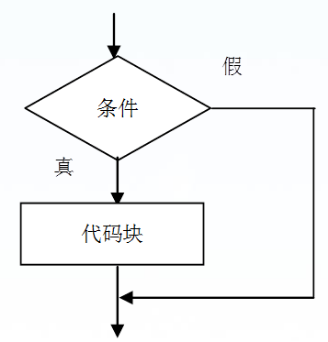if ( 条件 ) {
//代码块
}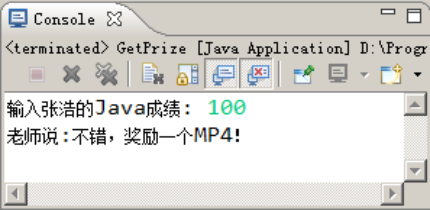import java.util.Scanner;
public class GetPrize {
public static void main(String[] args) {
Scanner input = new Scanner(System.in);
System.out.print("输入张浩的Java成绩: "); //提示输入Java成绩
int score = input.nextInt(); //从控制台获取张浩的Java成绩
if ( score > 90 ) { //判断是否大于90分
System.out.println(“老师说:不错，奖励一个MP4！”);
}
}
}
2.if…else if…else 结构

if(布尔表达式 1){
//如果布尔表达式 1的值为true执行代码
}else if(布尔表达式 2){
//如果布尔表达式 2的值为true执行代码
}else if(布尔表达式 3){
//如果布尔表达式 3的值为true执行代码
}else {
//如果以上布尔表达式都不为true执行代码
}

public class Test {
public static void main(String args[]){
int x = 30;

if( x == 10 ){
System.out.print("Value of X is 10");
}else if( x == 20 ){
System.out.print("Value of X is 20");
}else if( x == 30 ){
System.out.print("Value of X is 30");
}else{
System.out.print("这是 else 语句");
}

}
}
3.嵌套if

if(布尔表达式 1){

if(布尔表达式 2){

}
}

public class Test {

public static void main(String args[]){
int x = 30;
int y = 10;

if( x == 30 ){
if( y == 10 ){
System.out.print("X = 30 and Y = 10");
}
}
}

}

2.5.2 switch case
switch case 语句判断一个变量与一系列值中某个值是否相等，每个值称为一个分支。
switch case 语句语法格式如下：
switch(expression){
case value :
//语句
break; //可选
case value :
//语句
break; //可选
//你可以有任意数量的case语句
default : //可选
//语句
}

switch case 执行时，一定会先进行匹配，匹配成功返回当前 case 的值，再根据是否有 break，判断是否继续输出，或是跳出判断。

public class Test {
public static void main(String args[]){

{
case 'A' :
System.out.println("优秀");
break;
case 'B' :
case 'C' :
System.out.println("良好");
break;
case 'D' :
System.out.println("及格");
break;
case 'F' :
System.out.println("你需要再努力努力");
break;
default :
System.out.println("未知等级");
}

}
}

public class Test {
public static void main(String args[]){
int i = 5;
switch(i){
case 0:
System.out.println(“0”);
case 1:
System.out.println(“1”);
case 2:
System.out.println(“2”);
default:
System.out.println(“default”);
}
}
}

default

public class Test {
public static void main(String args[]){
int i = 1;
switch(i){
case 0:
System.out.println(“0”);
case 1:
System.out.println(“1”);
case 2:
System.out.println(“2”);
default:
System.out.println(“default”);
}
}
}

1
2
default

2.6 循环控制语句
2.6.1 while/do while
1.while
while是最基本的循环，它的结构为：
while( 布尔表达式 ) {
//循环内容
}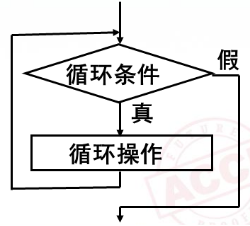public class Test {
public static void main(String args[]) {
int x = 10;
while( x < 20 ) {
System.out.print(“value of x : " + x );
x++;
System.out.print(”\n");
}
}
}

value of x : 10
value of x : 11
value of x : 12
value of x : 13
value of x : 14
value of x : 15
value of x : 16
value of x : 17
value of x : 18
value of x : 19

2.do while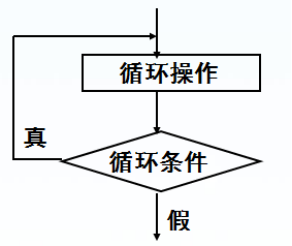do…while 循环和 while 循环相似，不同的是，do…while 循环至少会执行一次。
do…while 循环的结构如下：
do {
//代码语句
}while(布尔表达式);

public class Test {
public static void main(String args[]){
int x = 10;

do{
System.out.print("value of x : " + x );
x++;
System.out.print("\n");
}while( x < 20 );

}
}

value of x : 10
value of x : 11
value of x : 12
value of x : 13
value of x : 14
value of x : 15
value of x : 16
value of x : 17
value of x : 18
value of x : 19
2.6.2 for
Java 提供了另一种语句 —— for 循环，使一些循环结构变得更加简单。for循环执行的次数是在执行前就确定的。语法格式如下：
for循环的语法和执行顺序：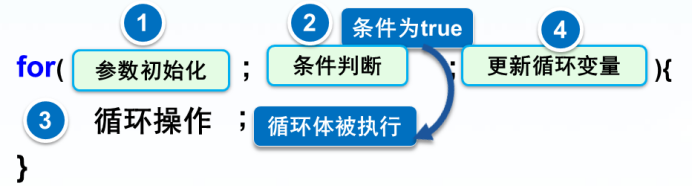int i=0;
while(i<100){
System.out.println(“好好学习！”);
i++;
}

for(int i=0;i<100;i++){
System.out.println(“好好学习！”);
}
2.6.3 break和continue
1.break

for (int i = 0; i<10; i++) {
//跑4000米；
if ( 不能坚持 ) {
break; //退出比赛
}
}

break用于do-while、while、for中时，可跳出循环而执行循环后面的语句。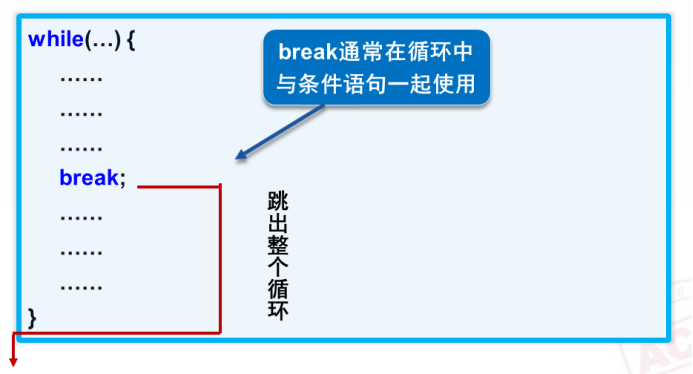public class Test {
public static void main(String args[]) {
int [] numbers = {10, 20, 30, 40, 50};

for(int x : numbers ) {
// x 等于 30 时跳出循环
if( x == 30 ) {
break;
}
System.out.print( x );
System.out.print("\n");
}

}
}

10
20

2.continue

continue 适用于任何循环控制结构中。作用是跳过循环体中剩余的语句而执行下一次循环。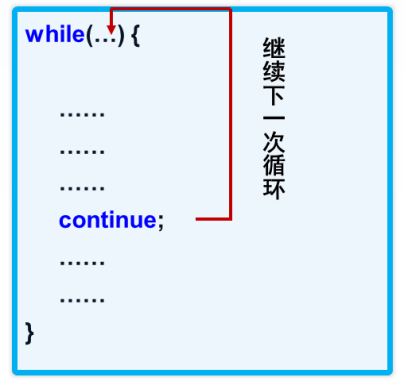import java.util.Scanner;
public class ContinueDemo {
/**
* 统计80分以上学生比例
*/
public static void main(String[] args) {
int score; // 成绩
int total; // 班级总人数
int num = 0; // 成绩大于或等于80分的人数
Scanner input = new Scanner(System.in);
System.out.print("输入班级总人数: ");
total = input.nextInt(); // 输入班级总数
for (int i = 0; i < total; i++) {
System.out.print(“请输入第” + (i + 1) + "位学生的成绩： ");
score = input.nextInt();
if (score < 80) {
continue;
}
num++;
}
System.out.println("80分以上的学生人数是： " + num);
double rate = (double) num / total * 100;
System.out.println(“80分以上的学生所占的比例为：” + rate + “%”);
}
}

07-261990
10-201万+03-0588
07-24120
11-04167
01-201212
04-147560
11-01189
08-21180
03-0523
02-1526
12-17560
10-17251
07-23942
08-05205
01-144505
02-26310
05-2927
10-302573qq_49622608

¥2 ¥4 ¥6 ¥10 ¥20余额支付 (余额：-- )扫码支付获取中扫码支付点击重新获取扫码支付1.余额是钱包充值的虚拟货币，按照1:1的比例进行支付金额的抵扣。
2.余额无法直接购买下载，可以购买VIP、C币套餐、付费专栏及课程。余额充值